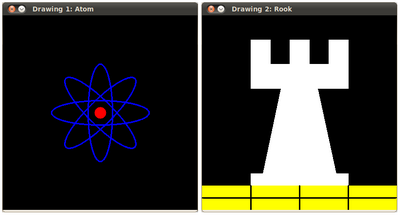OpenCV  4.8.0-dev Open Source Computer Vision
Basic Drawing

Next Tutorial: Random generator and text with OpenCV

Original author Ana Huamán
Compatibility OpenCV >= 3.0

## Goals

In this tutorial you will learn how to:

• Draw a line by using the OpenCV function line()
• Draw an ellipse by using the OpenCV function ellipse()
• Draw a rectangle by using the OpenCV function rectangle()
• Draw a circle by using the OpenCV function circle()
• Draw a filled polygon by using the OpenCV function fillPoly()

## Explanation

Since we plan to draw two examples (an atom and a rook), we have to create two images and two windows to display them.

We created functions to draw different geometric shapes. For instance, to draw the atom we used MyEllipse and MyFilledCircle:

And to draw the rook we employed MyLine, rectangle and a MyPolygon:

Let's check what is inside each of these functions:

#### MyLine

• As we can see, MyLine just call the function line() , which does the following:
• Draw a line from Point start to Point end
• The line is displayed in the image img
• The line color is defined by ( 0, 0, 0 ) which is the RGB value correspondent to Black
• The line thickness is set to thickness (in this case 2)
• The line is a 8-connected one (lineType = 8)

#### MyEllipse

• From the code above, we can observe that the function ellipse() draws an ellipse such that:
• The ellipse is displayed in the image img
• The ellipse center is located in the point (w/2, w/2) and is enclosed in a box of size (w/4, w/16)
• The ellipse is rotated angle degrees
• The ellipse extends an arc between 0 and 360 degrees
• The color of the figure will be ( 255, 0, 0 ) which means blue in BGR value.
• The ellipse's thickness is 2.

#### MyFilledCircle

• Similar to the ellipse function, we can observe that circle receives as arguments:
• The image where the circle will be displayed (img)
• The center of the circle denoted as the point center
• The radius of the circle: w/32
• The color of the circle: ( 0, 0, 255 ) which means Red in BGR
• Since thickness = -1, the circle will be drawn filled.

#### MyPolygon

• To draw a filled polygon we use the function fillPoly() . We note that:
• The polygon will be drawn on img
• The vertices of the polygon are the set of points in ppt
• The color of the polygon is defined by ( 255, 255, 255 ), which is the BGR value for white

#### rectangle

• Finally we have the cv::rectangle function (we did not create a special function for this guy). We note that:
• The rectangle will be drawn on rook_image
• Two opposite vertices of the rectangle are defined by ( 0, 7*w/8 ) and ( w, w )
• The color of the rectangle is given by ( 0, 255, 255 ) which is the BGR value for yellow
• Since the thickness value is given by FILLED (-1), the rectangle will be filled.

## Result

Compiling and running your program should give you a result like this: